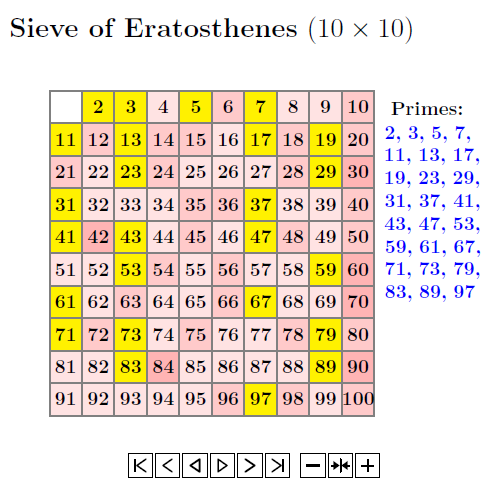# 1.3: Primes

$$\newcommand{\vecs}{\overset { \rightharpoonup} {\mathbf{#1}} }$$ $$\newcommand{\vecd}{\overset{-\!-\!\rightharpoonup}{\vphantom{a}\smash {#1}}}$$$$\newcommand{\id}{\mathrm{id}}$$ $$\newcommand{\Span}{\mathrm{span}}$$ $$\newcommand{\kernel}{\mathrm{null}\,}$$ $$\newcommand{\range}{\mathrm{range}\,}$$ $$\newcommand{\RealPart}{\mathrm{Re}}$$ $$\newcommand{\ImaginaryPart}{\mathrm{Im}}$$ $$\newcommand{\Argument}{\mathrm{Arg}}$$ $$\newcommand{\norm}{\| #1 \|}$$ $$\newcommand{\inner}{\langle #1, #2 \rangle}$$ $$\newcommand{\Span}{\mathrm{span}}$$ $$\newcommand{\id}{\mathrm{id}}$$ $$\newcommand{\Span}{\mathrm{span}}$$ $$\newcommand{\kernel}{\mathrm{null}\,}$$ $$\newcommand{\range}{\mathrm{range}\,}$$ $$\newcommand{\RealPart}{\mathrm{Re}}$$ $$\newcommand{\ImaginaryPart}{\mathrm{Im}}$$ $$\newcommand{\Argument}{\mathrm{Arg}}$$ $$\newcommand{\norm}{\| #1 \|}$$ $$\newcommand{\inner}{\langle #1, #2 \rangle}$$ $$\newcommand{\Span}{\mathrm{span}}$$$$\newcommand{\AA}{\unicode[.8,0]{x212B}}$$

Definition:

Prime Numbers - integers greater than $$1$$ with exactly $$2$$ positive divisors: $$1$$ and itself.
Let $$n$$ be a positive integer greater than $$1$$. Then $$n$$ is called a prime number if $$n$$ has exactly two positive divisors, $$1$$ and $$n.$$

Composite Numbers - integers greater than 1 which are not prime.

Note that: $$1$$ is neither prime nor composite.

There are infinitely many primes, which was proved by Euclid in 100BC.

Example $$\PageIndex{1}$$: Method of Sieve of Eratosthenes

Examples of prime numbers are $$2$$ (this is the only even prime number), $$3, 5, 7, 9, 11, 13, 17, \ldots$$.

Method of Sieve of Eratosthenes:The following will provide us a way to decide given number is prime.

Theorem $$\PageIndex{1}$$

Let $$n$$ be a composite number with exactly $$3$$ positive divisors. Then there exists a prime $$p$$ such that $$n=p^2.$$

Proof

Let $$n$$ be a positive integer with exactly $$3$$ positive divisors. Then two of the positive divisors are $$1$$ and $$n.$$
Let $$d$$ be the third positive divisor. Then $$1 < d < n$$ and the only positive divisors of $$d$$ are $$1$$ and $$d.$$
Therefore $$d$$ is prime.
Since $$d \mid n$$, $$n=md, m \in\mathbb{Z_+}$$.
Since $$m \mid n$$ and $$1 <m<n, m=d, n=d^2.$$
Thus, $$d$$ is prime. $$\Box$$

Theorem $$\PageIndex{2}$$

Every composite number $$n$$ has a prime divisor less than or equal to $$\sqrt{n}$$. If $$p$$ is a prime number and $$p \mid n$$, then $$1<p \leq \sqrt{n}.$$

Proof

Suppose $$p$$ be the smallest prime divisor of $$n$$. Then there exists an integer $$m$$ such that $$n=pm$$. Assume that $$p > \sqrt{n}$$. Then $$m < \sqrt{n}.$$

Let $$q$$ be any prime divisor of $$m$$. Then $$q$$ is also a prime divisor of $$n$$ and $$q < m < \sqrt{n} < p.$$ This is a contradiction.

Example $$\PageIndex{2}$$:

Is $$223$$ a prime number?

Solution:

The prime numbers $$< \sqrt{223}$$ are as follows: $$2, 3, 5, 7, 11,$$ and $$13$$. Note that $$17^2 >223.$$

Since $$223$$ is not divisible by any of the prime numbers identified above, $$223$$ is a prime number.

Exercise $$\PageIndex{3}$$

Is $$2011$$ a prime number?.

yes.

Theorem $$\PageIndex{3}$$: Prime divisibility theorem

Let $$p$$ be a prime number. If $$p \mid ab, a,b \in \mathbb{Z},$$ then $$p\mid a$$ or $$p\mid b$$.

Proof

Let $$p$$ be a prime number and let $$a,b \in \mathbb{Z}.$$  Assume that $$p \mid ab$$. If $$p\mid a$$, we are done. So we suppose that $$p$$ does not divide $$a$$. Thus $$gcd(a,p)=1.$$ Thus there exists $$x,y \in \mathbb{Z}$$ such that $$1=ax+py$$. Hence $$b=abx+pby$$. Since $$p \mid ab$$, $$ab=pm, m\in \mathbb{Z}.$$ Thus $$b=pmx+pby=p(mx+by).$$ Hence, $$p\mid b.$$

Theorem $$\PageIndex{5}$$

There are infinitely many primes.

Proof

Suppose there are finitely many primes $$p_1, p_2, ...,p_n$$, where $$n$$ is a positive integer. Consider the integer $$Q$$ such that

$Q=p_1p_2...p_n+1.$

Notice that $$Q$$ is not prime. Hence $$Q$$ has at least a prime divisor, say $$q$$. If we prove that $$q$$ is not one of the primes listed then we obtain a contradiction. Suppose now that $$q=p_i$$ for $$1\leq i\leq n$$. Thus $$q$$ divides $$p_1p_2...p_n$$ and as a result $$q$$ divides $$Q-p_1p_2...p_n$$. Therefore $$q$$ divides 1. But this is impossible since there is no prime that divides 1 and as a result $$q$$ is not one of the primes listed.

The following theorem explains the gaps between prime numbers:

Theorem $$\PageIndex{5}$$

Let $$n$$ be an integer. Then there exists $$n$$ consecutive composite integers.

Proof

Let $$n$$ be an integer. Consider the sequence of integers $(n+1)!+2, (n+1)!+3,...,(n+1)!+n, (n+1)!+(n+1)$

Notice that every integer in the above sequence is composite because $$k$$ divides $$(n+1)!+k$$ if $$2\leq k\leq (n+1)$$.

Example $$\PageIndex{4}$$:

Does there exist a block of $$1000000$$ consecutive integers without a prime number among them?

Solution

Use $$1000001 !$$ and consider $$1000001 ! +2 , \ldots, 1000001!+1000001$$

Example $$\PageIndex{5}$$:

Consider the formula $$y=x^2+x+13$$. Then

1. Find the values $$y$$ for $$x=1, \cdots, 12$$. How many of these values are prime?

2. Can we conclude that this formula always generates a prime number?

Definition: Twin primes

Two prime numbers are called twin primes if they differ by $$2$$.

Theorem $$\PageIndex{6}$$ Fundamental Theorem of Arithmetic (FTA)

Let $$n \in\mathbb{Z_+}$$, then n can be expressed as a product of primes in a unique way. This means

$$n=p_1^{n_1} p_2^{n_2} \cdots p_k^{n_k}$$, where $$p_1 <p_2< ... <p_k$$ are primes.

Neither the fundamental theorem nor the proof shows us how to find the prime factors. We can use tests for divisibility to find prime factors whenever possible.

Example $$\PageIndex{6}$$:

Find the prime factorization of

1. $$252$$

2.$$2018$$.

3. $$1000$$

Solution

1. Using divisibility tests, we can find that $$252= 2^2 3^2 7$$

2. We can see that $$2018$$ is divisible by 2, and $$2018= 2 \times 1009$$. $$1009$$ is prime (why?).

3. $$1000=10^3=((2)(5))^3=2^3 5^3$$

Example $$\PageIndex{7}$$:

Find the prime factorization of $$1000001$$.

Solution

Consider $$1000001=1000000+1= 10^6+1=(10^2)^3+1^3= (10^2+1^2) (10^4-10^2+1^2)= 101 \times 9901$$. $$101$$ and $$9901$$ are prime (why?)

Note: Conjectures

Goldbach's Conjecture(1742)

Every even integer $$n >2$$ is the sum of two primes.

Goldbach's Ternary Conjecture:

Every odd integer $$n \geq 5$$ is the sum of at most three primes.

This page titled 1.3: Primes is shared under a CC BY-NC-SA 4.0 license and was authored, remixed, and/or curated by Pamini Thangarajah.